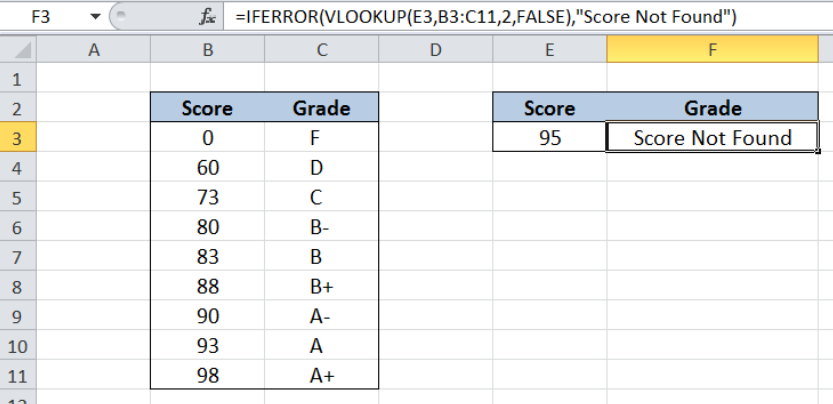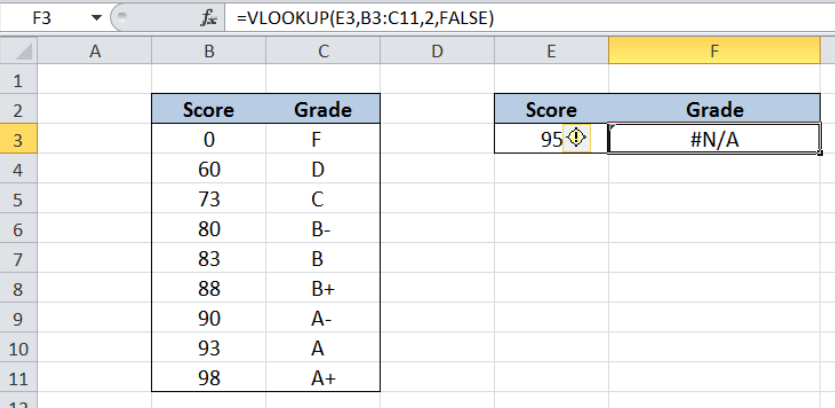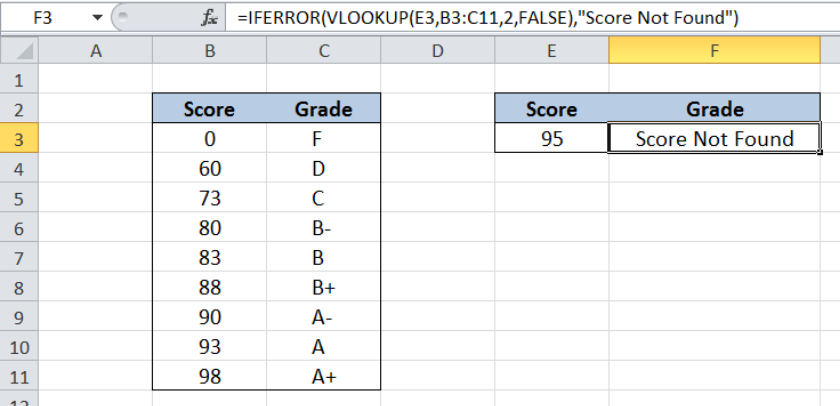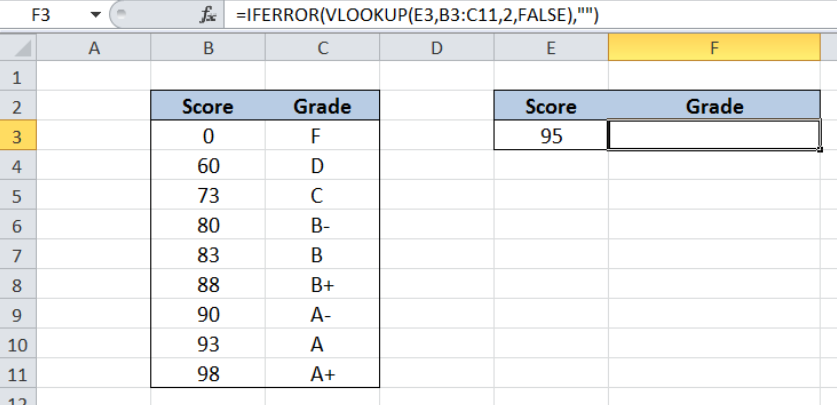Get instant live expert help with Excel or Google Sheets“My Excelchat expert helped me in less than 20 minutes, saving me what would have been 5 hours of work!”

#### Post your problem and you’ll get Expert help in seconds.

Your message must be at least 40 characters
Our professional Expert are available now. Your privacy is guaranteed.

# VLOOKUP without #N/A error

VLOOKUP provides a quick way of looking up a value from any list or range.  However, if a value is not found, VLOOKUP returns an error value #N/A. This step by step tutorial will assist all levels of Excel users in returning a custom value instead of error values by incorporating the IFERROR in the VLOOKUP function.Figure 1. Final result: VLOOKUP without #N/A error

Final formula:  `=IFERROR(VLOOKUP(E3,B3:C11,2,FALSE),"Score Not Found")`

## Syntax of VLOOKUP function

`=VLOOKUP(lookup_value, table_array, col_index_num, [range_lookup])`

The parameters of the VLOOKUP function are:

• lookup_value – the value that we want to search and find in the table_array
• table_array – the range of cells in the source table containing the data we want to retrieve
• col_index_num the column number in the table_array corresponding to the information we want to retrieve, relative to the lookup_value
• [range_lookup] – optional; value is either TRUE or FALSE
• if TRUE or omitted, VLOOKUP returns either an exact or approximate match
• if FALSE, VLOOKUP will only find an exact match

## Syntax of IFERROR Function

IFERROR function evaluates if a formula results to an error and returns a specified value; otherwise, it returns the result of the formula being evaluated

`=IFERROR(value, value_if_error)`

• value – the value or formula we want to check for an error
• value_if_error  – the value that IFERROR returns if the value or formula results to an error.
• IFERROR evaluates the following error types: #N/A, #VALUE!, #REF!, #DIV/0!, #NUM!, #NAME?, or #NULL!

## Setting up Our Data

Our data consists of two columns:  Score (column B) and Grade (column C).  In cell E3, we enter the score we want to look up from our data in B3:C11.  In cell F3, we want to determine the corresponding grade of “95” by using the VLOOKUP function.  The formula we use is:

`=VLOOKUP(E3,B3:C11,2,FALSE)`

As shown below, the value in cell F3 is an error value #N/A because the score “95” is not found in the table B3:C11.Figure 2. Sample data  to hide #N/A error in VLOOKUP

We want to hide the error value that VLOOKUP returns when it cannot found a value.

## VLOOKUP without #N/A error

In order to hide the error that VLOOKUP returns and instead show our custom value, we incorporate the IFERROR function into our VLOOKUP formula.  Let us follow these steps:

Step 1.  Select cell F3

Step 2.  Enter the formula: `=``IFERROR(VLOOKUP(E3,B3:C11,2,FALSE),"Score Not Found")`

Step 3:  Press ENTERFigure 3.  IFERROR returns custom value instead of error values in VLOOKUP

The IFERROR function evaluates our VLOOKUP formula if it returns an error.   In our example, we want to find the score 95, but it is not found in the table B3:C11.  The VLOOKUP returns the #N/A error.  Hence, IFERROR evaluates TRUE and returns the value that we want to show in case of errors: “Score Not Found”.

As a result, the value in cell F3 is changed from the #N/A error to the text string we want to display which is “Score Not Found”.

While using the IFERROR function, we can also show an empty string instead of displaying the error values.  We can do this by entering this formula in cell F3:

`=IFERROR(VLOOKUP(E3,B3:C11,2,FALSE),"")`Figure 4. Output: IFERROR returns an empty string instead of error values in VLOOKUP

Most of the time, the problem you will need to solve will be more complex than a simple application of a formula or function. If you want to save hours of research and frustration, try our live Excelchat service! Our Excel Experts are available 24/7 to answer any Excel question you may have. We guarantee a connection within 30 seconds and a customized solution within 20 minutes.

### Did this post not answer your question? Get a solution from connecting with the expert.Another blog reader asked this question today on Excelchat:
Solution examplesI'm looking for a formula or format for if there is data in c4 on a sheet... It adds extra information underneath
Solved by X. E. in 20 minshi, my vlookup wont work in some rows but does in others. its temperemental. e.g it will work on rows 10 to 15, then not row 16 and 17, but the will for the rest.
Solved by K. Q. in 21 minshow to make a format that if multiple cells have number contents its value is 1 in the last cell
Solved by C. C. in 28 minsMy VLOOKUP formula won't work when value the "lookup cell" is derived from a formula. If I manually transfer the formula derived value to a different cell, then VLOOKUP formula works fine. What's going on?
Solved by Z. J. in 40 minsI need to find out if the 2018 yearly sales goals were met if the yearly sales were \$25,000 or more using an IF logical function and to set the formula to return a value of YES if met, and NO if not
Solved by A. A. in 18 mins## Subscribe to Excelchat.coAnother blog reader asked this question today on Excelchat: